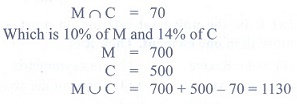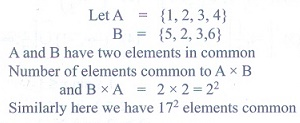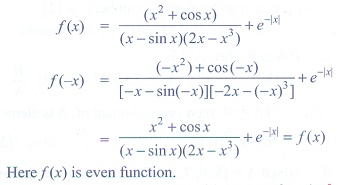Home | | Maths 11th std | Exercise 1.5: Choose the correct or the most suitable answer

# Exercise 1.5: Choose the correct or the most suitable answer

Mathematics : Sets, Relations and Functions: Multiple choice questions with answers / choose the correct answer with answers - Maths Book back 1 mark questions and answers with solution for Exercise Problems

CHAPTER: Sets, Relations and Functions

Choose the correct or the most suitable answer.

1. If A = {(x, y) : y = ex, xR} and B = {(x, y) : y = e-x, x  R} then n(A  B) is

(1) Infinity

(2) 0

(3) 1

(4) 2

Solution2. If A = {(x, y) : y = sin x, x  R} and B = {(x, y) : y = cos x, x  R} then A  B contains

(1) no element

(2) infinitely many elements

(3) only one element

(4) cannot be determined.

3. The relation R defined on a set A = {0,-1, 1, 2} by xRy if |x2 + y2| ≤  2, then which one of the following is true?

(1) R = {(0, 0), (0,-1), (0, 1), (-1, 0), (-1, 1), (1, 2), (1, 0)}

(2) R-1 = {(0, 0), (0,-1), (0, 1), (-1, 0), (1, 0)}

(3) Domain of R is {0,-1, 1, 2}

(4) Range of R is {0,-1, 1}

Solution4. If {(x) = |x  2| + |x + 2|, x ∊ R, thenAns: 1

Solution5. Let R be the set of all real numbers. Consider the following subsets of the plane R x  R:

S = {(x, y) : y = x + 1 and 0 < x < 2} and T = {(x, y) : x - y is an integer }

Then which of the following is true?

(1) T is an equivalence relation but S is not an equivalence relation.

(2) Neither S nor T is an equivalence relation

(3) Both S and T are equivalence relation

(4) S is an equivalence relation but T is not an equivalence relation.

Solution6. Let A and B be subsets of the universal set N, the set of natural numbers. Then A’ [(AB)B’] is

(1) A

(2) A’

(3) B

(4) N

Solution7. The number of students who take both the subjects Mathematics and Chemistry is 70. This

represents 10% of the enrollment in Mathematics and 14% of the enrollment in Chemistry. The

number of students take at least one of these two subjects, is

(1) 1120

(2) 1130

(3) 1100

(4) insufficient data

Solution8. If n((A x B)  (A x C)) = 8 and n(B  C) = 2, then n(A) is

(1) 6

(2) 4

(3) 8

(4) 16

Solution9. If n(A) = 2 and n(B C) = 3, then n[(A x B)  (A xC)] is

(1) 23

(2) 32

(3) 6

(4) 5

Solution10. If two sets A and B have 17 elements in common, then the number of elements common to the

set A x B and B x A is

(1) 217

(2) 172

(3) 34

(4) insufficient data

Solution11. For non-empty sets A and B, if A  B then (A x B)  (B x A) is equal to

(1) A  B

(2) A x A

(3) B x B

(4) none of these.

Solution12. The number of relations on a set containing 3 elements is

(1) 9

(2) 81

(3) 512

(4) 1024

Solution

Let S = {a,b,c}

n(S) = 3

n(S x S) = 9

Number of relations in n{P(SxS)} = 29  = 512

13. Let R be the universal relation on a set X with more than one element. Then R is

(1) not reflexive

(2) not symmetric

(3) transitive

(4) none of the above

Solution

Let X ={a,b,c}

Then R = Universal relation

= {(a,a), (a,b)(a,c)(b,a), (b,b)(b,c)(c,a), (c,b)(c,c)}

14. Let X = {1, 2, 3, 4} and R = {(1, 1), (1, 2), (1, 3), (2, 2), (3, 3), (2, 1), (3, 1), (1, 4), (4, 1)}. Then

R is

(1) reflexive

(2) symmetric

(3) transitive

(4) equivalence

Solution15. The range of the function 1 / 1-2 sin x isAns: 4

Solution16. The range of the function f(x) = |└ x┘- x| , x  R is

(1) [0, 1]

(2) [0, ∞)

(3) [0, 1)

(4) (0, 1)

Solution17. The rule f(x) = x2 is a bijection if the domain and the co-domain are given by

(1) R,R

(2) R, (0, ∞)

(3) (0, ∞),R

(4) [0, ∞), [0, ∞)

Solution18. The number of constant functions from a set containing m elements to a set containing n elements

is

(1) mn

(2) m

(3) n

(4) m + n

19. The function f : [0, 2π] → [-1, 1] defined by f(x) = sin x is

(1) one-to-one

(2) onto

(3) bijection

(4) cannot be defined

Solution20. If the function f : [-3, 3] → S defined by f(x) = x2 is onto, then S is

(1) [-9, 9]

(2) R

(3) [-3, 3]

(4) [0, 9]

Solution21. Let X = {1, 2, 3, 4}, Y = {a, b, c, d} and f = {(1, a), (4, b), (2, c), (3, d), (2, d)}. Then f is

(1) an one-to-one function

(2) an onto function

(3) a function which is not one-to-one

(4) not a function

Solution22. The inverse ofAns: 1

Solution23. Let f : R → R be defined by f(x) = 1 - |x|. Then the range of f is

(1) R

(2) (1,∞)

(3) (-1, ∞)

(4) (-∞, 1]

Solution24. The function f : R → R is defined by f(x) = sin x + cos x is

(1) an odd function

(2) neither an odd function nor an even function

(3) an even function

(4) both odd function and even function.

Solution25. The function f : R → R is defined byis

(1) an odd function

(2) neither an odd function nor an even function

(3) an even function

(4) both odd function and even function.

SolutionTags : Sets, Relations and Functions | Mathematics , 11th Mathematics : UNIT 1 : Sets, Relations and Functions
Study Material, Lecturing Notes, Assignment, Reference, Wiki description explanation, brief detail
11th Mathematics : UNIT 1 : Sets, Relations and Functions : Exercise 1.5: Choose the correct or the most suitable answer | Sets, Relations and Functions | Mathematics